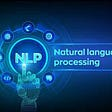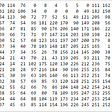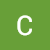# Checklist for training Deep Learning Model

Here are a few checklists which one can follow while training Deep Learning Models.

## 1. Preprocess the data.

For numeric data, you can scale or normalize. For text data, we can remove stop words, tokenize them, convert them to vector representation using TFIDF, BoW, Word2Vec, etc.

## 2. Initialize the weights appropriately.

There are a few weight initialization techniques.

a. Xavier / Glorot initializer. We can use normal or uniform. This is preferably used when we have sigmoid or tanh as an activation unit.

b. He initializer. Here as well we have normal and uniform. his is preferably used when we have ReLU activation unit.

c. Random Normal initializer. We can pass mean and standard deviation as a parameter along with the seed.

d. Random Uniform initializer. We can pass minimum value and maximum value as a parameter along with the seed.

e. Zero initialization. Please avoid it.

For more on weight, initialization refers to this.

## 3. Choose the proper activation function.

As of now, ReLu is the most popular activation function which is widely used in most layers apart from the output layer. There are many variations of ReLu.

We need to choose the activation function depending on the problem statement for the output layer. For binary classification, we can use Sigmoid and for multiclass classification, we can use Softmax.

For the regression problem, we can choose linear activation. Linear activation also called “Passthrough” because here output is the same as the input.

## 4. Avoid the overfitting of the model.

There are a lot of parameters in the Deep Learning model. Due to this model can easily overfit. To avoid overfitting and faster convergence we can add the BatchNormalization layer (We batch normalize Z, not X, where Z is W Transpose X) and/or Dropout layer. Dropout is one of the best regularization techniques for the Deep Learning Model. These are generally added to the later layers which are closer to the output.

## 5. Choose proper Optimizer.

Adam is one of the popular Optimizer but depending on data and problem statement we can choose different Optimizer.

## 6. Choose hyperparameters wisely

Deep Learning models have a lot of Hyperparameters as Layers, Units in each layer, Dropout rate, Kernel size, Stride, Pool Size, Learning rate, etc.

## 7. Choose Loss function depending on the problem statement.

For Binary classification we can use BinaryCrossentropy. For Multiclass classification, we can use CategoricalCrossentropy. Dice Loss is used when we need to do a pixel-wise comparison of images. For the regression problem, we can use MSE, MAE, etc.

## 8. Always monitor the weights (Gradient Checking and Clipping).

To detect problems like Vanishing Gradient or Exploding Gradient we should monitor weight. This can be done by using Tensorboard.

The solution to the Exploding Gradient is Gradient Clipping. Clipping gets the values into a scale where the value will always be less than or equal to the clip value.

In the default case where all dimensions are used for calculation, if the L2-norm of “W” is already less than or equal to “threshold”, then “W” is not modified. If the L2-norm is greater than “threshold”, then this operation returns a tensor of the same type and shape as “W” with its values set to:

W = (W * threshold) / l2_norm(W)

## 9. Plots.

Very important to make sense of the model performance. The model is good when Train and Test loss are closer.

## 10. Use callbacks.

There are different callbacks for different purposes. The callback which I used extensively is ModelCheckpoint, TensorBoard, ReduceLROnPlateau, LearningRateScheduler, TerminateOnNaN.

# Reference

--

--

--

## More from Chinmayi Sahu

Machine Learning Enthusiast

Love podcasts or audiobooks? Learn on the go with our new app.

## Distilling BERT — How to achieve BERT performance using logistic regression## Adopt a buddy (Part 2 of 3)## End to End NLP Pipeline## Introduction to Machine Learning## Defining Key Terms & Concepts in Neural Networks (Part 1)## Machine learning is all about math## Chinmayi Sahu

Machine Learning Enthusiast

## Starting a new machine learning project? User this 3-tier system to gau## What are the Differences Between Deep Learning and Machine Learning?## TensorFlow and DJL Machine Learning Pipeline